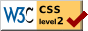# Rank Order as a Psychophysical Method

### Louis L. Thurstone University of Chicago

In every psychophysical experiment it is necessary for the investigator to select a psychophysical method and in doing so he is guided by two principal types of consideration, namely experimental convenience and theoretical propriety. Other things being equal, he will choose that experimental procedure which is simplest for the subject and most convenient. He will also be constrained to select a method which is legitimate for his problem. One of the simplest of experimental procedures ,is to ask the subject to arrange a series of stimuli in absolute rank order according to length, weight, brightness, beauty or whatever the psychological continuum may happen to be. This experimental procedure does not ordinarily lend itself to psychological measurement because the subjective increments represented by pie successive rank orders are ordinarily entirely unknown. Occasionally it is legitimate to assume that the entire distribution of subjective values is Gaussian and then it is possible to translate rank orders into legitimate psychological measurement. We are dealing now with the situation in which a small number of stimuli, such as ten or twenty, are to be dealt with and in which no assumption can be made legitimately regarding their distribution in subjective value.

For theoretical purposes the constant method is probably the best in most experimental problems. The ideal form of the constant method is the method of paired comparison in which not one but all of the stimuli serve as standards, but

( 188) the constant method is also one of the most laborious experimental methods. If there are twenty stimuli and if the method is to be complete there would be required 190 judgments in order to compare each stimulus with every other stimulus. This assumes that the constant method is used in complete form with every stimulus serving in turn as a standard. The method has serious limitations when only one or two of the stimuli are used as standards. We shall also assume that the intermediate category is excluded. If the reader insists on using the intermediate category of judgment in the constant method, this paper is of no interest to him.

Our present problem is to devise a plan whereby simple absolute rank order may be used as the experimental procedure with the advantages of the much more laborious constant method. Given the data for absolute rank order we shall extract the proportion of judgments "A is greater than B" for every possible pair of stimuli in the given series. These derived proportions will be used instead of the proportions that are obtained directly in the constant method. From these derived proportions the subjective separations between any pair of stimuli can then be readily calculated by the equation of comparative judgment. The method will be derived first theoretically and then we shall describe its empirical verification.

If a subject has placed four stimuli A B C D in the rank order B D A C it is possible to tabulate his various comparisons as though he had made them separately. If each of the four stimuli were to be compared with every other one in the series it would require six separate judgments, namely AB AC AD BC BD CD. If there are n stimuli in the series it would require n(n — 1) such judgments with counterbalanced order of presentation or half that many if counterbalanced order is disregarded. This would give only one judgment for each of the possible pairs of stimuli. Now if the four stimuli have been placed in the rank order B D A C by one subject, it is clear that six judgments may be extracted from this one rank order. Evidently the above rank order series is equi-

( 189) -valent to the judgments B > D, B > A, B > C, D > A, D > C, A > C. If a large number of subjects have arranged fifteen or twenty stimuli in rank order it is an almost prohibitive task to tabulate the separate judgments to which the single rank order is equivalent. However, it can be done by a shorter procedure.

Let there be n specimens in the series to be arranged in rank order by N subjects. Let A and B be two of these specimens and let al= frequency with which specimen A is placed in rank 1 by the N subjects, b1= frequency with which specimen B is placed in rank i by the N subjects, pal= proportion of the N subjects who place specimen A in rank 1, pbl = proportion who place specimen B in rank 1, and similarly for the other specimens and the other rank orders. See figure 1.Since these values of p may be regarded as probabilities, we have pb2 + pb3 + pb4 + . . . pbn = pb>1 = probability that any subject at random (or any one judgment of a single subject) will place B in a rank higher than rank 1.

Hence pa1 . Pb>1= probability that any subject, chosen at random, will place A in rank 1, and B in a higher rank. Similarly, pa2 . pb>2 = probability that a subject will place A in rank 2 and B in a higher rank.

In general, this product may be written pak . pb>k =

( 190) probability that A will be perceived in rank k and that B will be perceived in a rank higher than k.

Summing for all of the n ranks, we have Σ(pak pb>k) = probability that B will be perceived in a rank higher than that of A.

But we must also consider the possibility that the two specimens will be perceived as of practically equal or nearly equal rank. If it were possible for two specimens to be perceived in the same rank order, then pak pbk = probability that both specimens A and B will be perceived in the same rank order k. But we assume that the subject is asked to place all the n specimens in absolute rank order without any duplicate or tied ranks and hence it is experimentally impossible for the two specimens to be placed in the same rank order. We shall make the assumption that if the two specimens are perceived to be sufficiently nearly alike to warrant the same rank order, the probability p1a>b = .50, and the probability p1b>a = .50. The notation p1 refers to a single class interval. This is not quite correct because we know that if the two stimuli are slightly different in objective measurement then there will be a slight majority of correct judgments while the incorrect judgments will be in the minority.

This, of course, ignores the possible time and space errors. This is legitimate in dealing with rank order as an experimental method because the subject is given a series of stimuli or specimens to sort out into a rank order by his own devices. It is therefore a matter of chance which of any pair of stimuli is perceived first and whether it happens to be held to the right or to the left of the second stimulus. The subject has the privilege of revising his results and of looking at any and all of the specimens in any order and as many times as he may choose. The problem of constant errors may therefore be ignored.

However, if the two specimens differ slightly in psychological value so that A > B and if they are presented to a subject repeatedly or once to a group of subjects in counter-balanced order by the constant method, we should find that pa>b > .50. In other words, a slight majority would favor specimen A. The departure of the judgment pa>b from .50

( 191) will be small if the difference between A and B is small. Since in a rank order experiment we shall assume that n is as large as 10 or 15 or 20, the interval in value represented by one rank order is relatively small. In such situations and especially when the discriminal error is much larger than the interval represented by one rank order, our assumption is approximately correct, namely that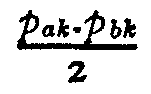= probability that both A and B will be perceived in the same rank order interval and that B will be
perceived higher than A.

Hence we may write the formula with this approximation as

pb>a = Σ(pak . pb>k) + ½ Σ (pak . pbk)(I)

In other words, we have expressed the proportion of subjects who perceive B higher than A in terms of the frequencies with which the two specimens are placed in the n rank orders. We can now use simple absolute rank order as an experimental procedure and we can obtain the same results as with the order of merit method and practically the same results as with the constant method with counterbalanced order. It is taken for granted here that the intermediate category is not used.

The approximation involved in the last term of equation (I) is close enough for all situations in which the number of stimuli to be arranged in rank order is greater than 10 or 15. The approximation is not satisfactory when the number of stimuli is small such as five or six. In the present study we have used the approximation represented by equation (I) but we shall develop here a more general formula for translating rank order into the proportions of the constant method which can be used when the experiment involves only a small number of stimuli.

If the number of stimuli represented in figure z is rather small it is evident that some distortion is introduced by regarding the probability ordinates to be constant within each. class interval. That is in effect the assumption in

( 192) deriving the approximation equation (1). That equation is derived as though the diagrams in figure 1 were drawn as column diagrams instead of probability polygons. Let figure 2 represent one of these class intervals in which the probabilities show variation within the class interval. The probability that stimulus i will be perceived in this class interval is p1, and the notation p2 has a similar interpretation for the second stimulus. The horizontal dotted lines in figure 2 represent the situation in which a stimulus would beas likely to be perceived at one part of the class interval as at any other part of it. Let the sloping straight line through pi represent for each value of x the probability that stimulus i will be perceived at x. As we have drawn figure 2, the

( 193) stimulus is more likely to be perceived in the upper part of the class interval than in the lower part of it but our correction formula will cover the general case in which the probability is assumed to vary throughout the class interval according to any linear function.

By inspection of figure 2 we see that the equations of the two sloping straight lines are as follows: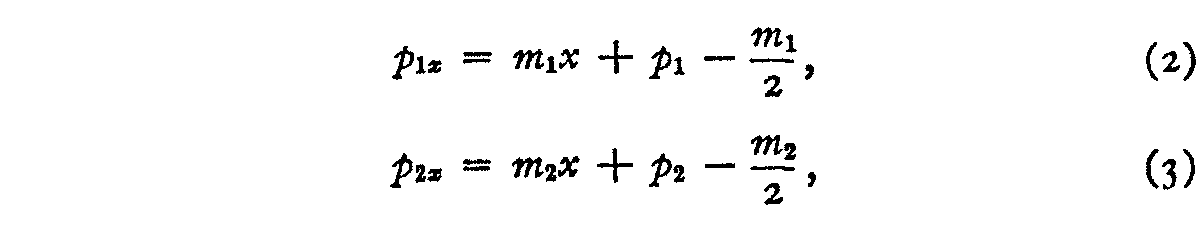in which

p1x = the probability that stimulus 1 will be perceived at any point x within the class interval.

p2x = the probability that stimulus 2 will be perceived at any point x within the class interval.

ml and m2 are the slopes of the lines.

pi and p2 are the probabilities that stimuli 1 and 2 respectively will be perceived in the class interval.

The probability P1x that stimulus 1 will be perceived higher than x but within the class interval is therefore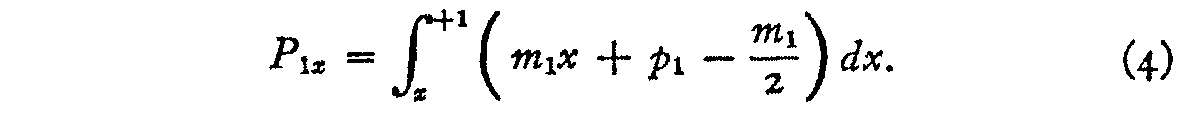The probability that stimulus 2 will be perceived at x and that stimulus i will be perceived higher than x but within the class interval is the product of these probabilities, namely,After integrating and simplifying, we have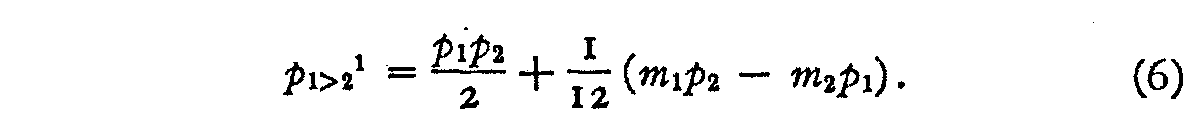( 194)

Inspection of equation (6) makes it evident that if the two slopes ml and m2 are zero, this term becomes identical with the second term of equation (1) which is what we should expect. Furthermore, if a and b are interchanged as well as p1 and p2 we should have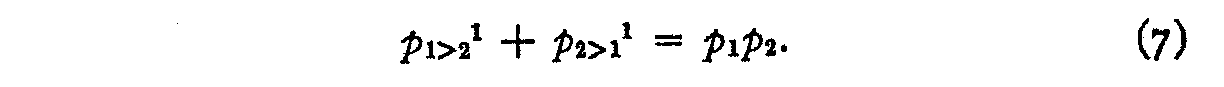In other words, the probability that both stimuli will be perceived in the same class interval is p1p2 . This probability is split into two parts, namely the probability p1>21 that 1 is perceived above 2, and the probability p2>11 that 2 is perceived above 1 in the same class interval.

If we use equation (6) in summation form instead of the second term of equation (1),we shall have a closer approximation to the true value of p1>2. Let the class interval of figure 2 be designated k and let the two stimuli 1 and 2 in figure 2 be designated b and a respectively, so that p2 and pi in that figure become, in the more general notation, pak and pbk respectively. Then the complete formula becomes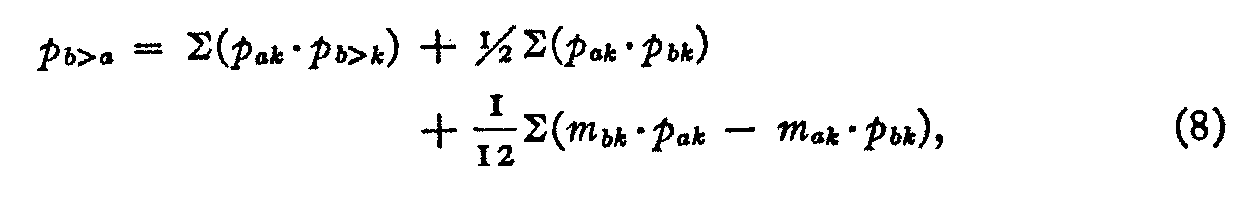in which the slopes mak and mbk are defined as follows:The notation in the general equation (8) may be summarized as follows :

pb>a = estimated proportion of subjects who judge stimulus b higher than stimulus a.

pak = proportion of subjects who place stimulus a in any particular rank order k. Similar interpretation for pbk.

pa>k = proportion of subjects who place stimulus a higher than any specified class interval k. Similar interpretation for pb>k.

( 195)

pa(k+1) = proportion of subjects who place stimulus a in the rank order next higher than k. Similar interpretation for pb(k+1)

pa(k-1) = proportion of subjects who place stimulus a in the rank order next lower thank. Similar interpretation for pb(k-1)

It should be noted that both of the equations (1) and (8) are approximation equations but that equation (8) involves the least assumptions. Thus equation (1) assumes that the probabilities in figure i can be adequately represented as column diagrams. This is legitimate for most problems where the number of stimuli is as large as, say 20. Equation (8) assumes only that the variation in the probabilities of each class interval in figure 2 is linear. It is represented by frequency polygons in figure 1 instead of column diagrams. In most experimental situations formula (1) is adequate since it shows only a very slight discrepancy with the actual count for pb>a.

Returning to formula (1) we shall now show its application to some experimental data. The psychophysical comparisons of social stimuli are much more complex than the comparisons of simple sensory stimuli such as line lengths and weights. If our method is applicable to the complexities of social stimuli, they may safely be assumed to be applicable to the simpler case of sensory stimuli. We shall test the formula on Miss Hevner's data for judgments about handwriting specimens.

In her experiment on the order of merit method she asked 370 subjects to arrange twenty specimens of handwriting in rank order. From such experimental data it was of course possible to count the number of subjects who placed each one of the twenty specimens in each one of the ?twenty rank orders. For example, 59 out of the 370 subjects placed specimen ¢ in the fifth place from the top in excellence. In a table of this kind there must of course be as many rank orders as there are specimens because tied ranks were not allowed. From this table a second table was prepared showing the

( 196)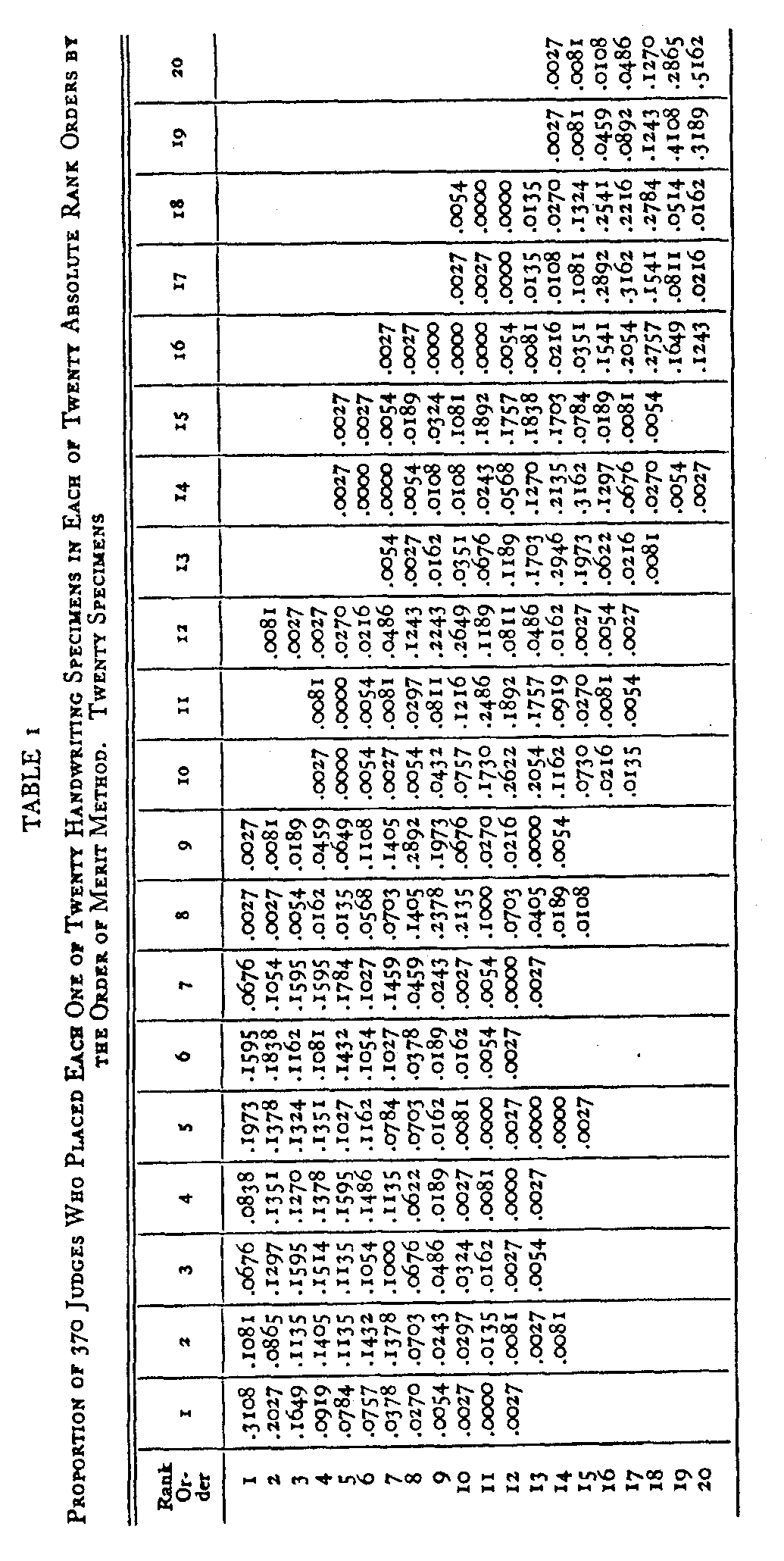( 197) proportion of all the subjects who placed each specimen in each of the twenty rank orders. In the above example this proportion is 0.1595. This means that about sixteen percent of the entire group of 370 subjects placed specimen 4 in rank order five. This is shown in Table 1 and the rest of the table is interpreted in the same manner.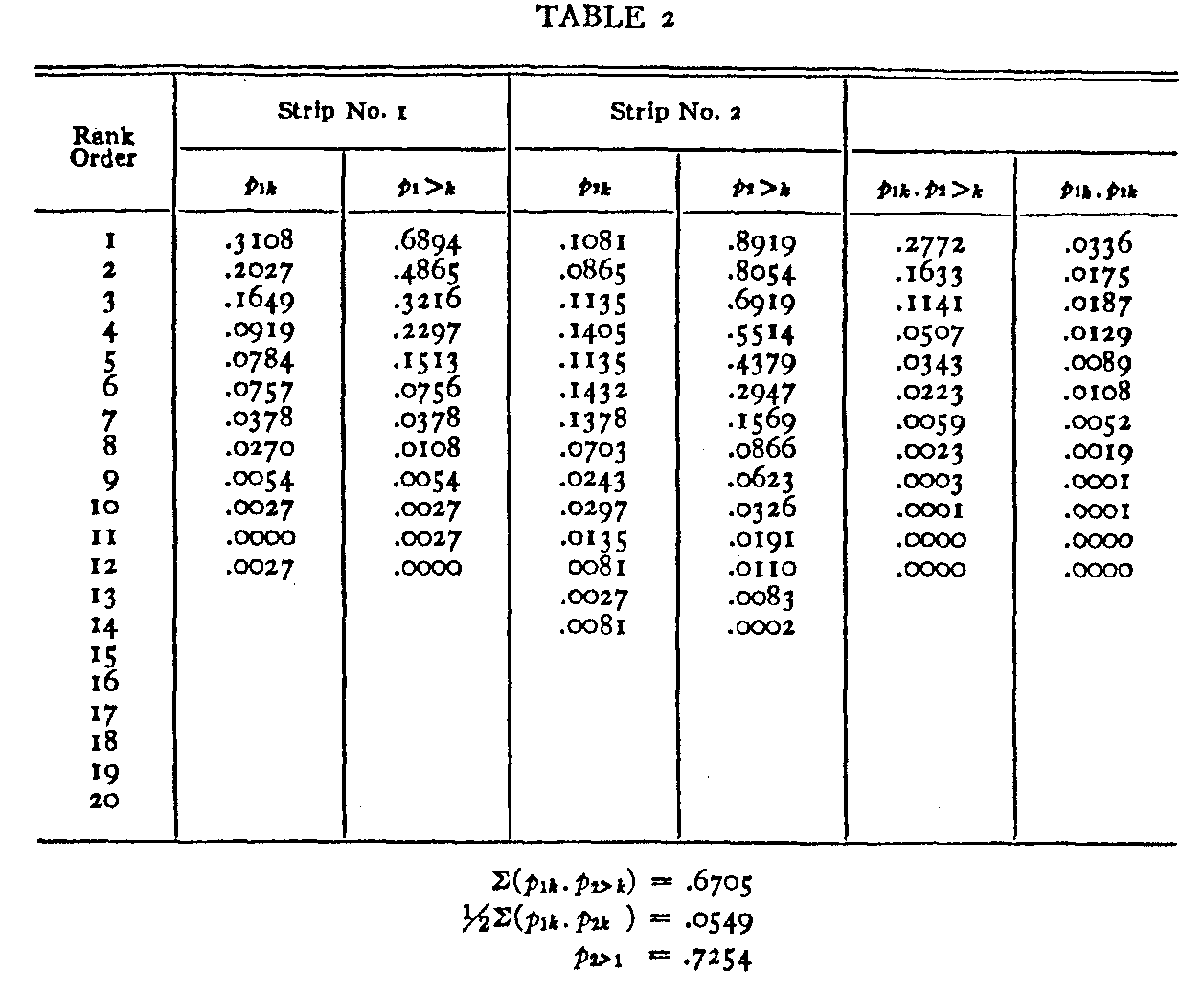Table 2 is a summary of the calculation for estimating the proportion of subjects who perceived specimen 2 to be better than specimen 1. The first column is a list of the twenty rank orders. For each specimen a strip was prepared similar to the second and third columns. Columns four and five in Table 2 represent such a strip for the second stimulus. These strips were used in calculation so as to avoid unnecessary transcription. The sixth column shows the product p1k .p2>k for each of the rank orders. This is merely the product of columns two and five in the table. The last column shows

( 198) the product p1k . p2k for each of the rank orders. It is the product of items in columns two and four. In actual calculation the entries in the last two columns were not recorded. The products were calculated on a Marchant calculating machine and they were allowed to total without recording of the separate items in the last two columns. The entries of these two columns are here shown for completeness al-though in practice it is not necessary to write them. Only the sums for the last two columns are recorded. These sums are shown at the bottom of the table. The simple calculation of the estimated proportion of all the subjects who perceived specimen 2 to be better than specimen i is also there indicated. It is done in accordance with equation z.

This procedure was carried out for each of the 1/2n(n — 1) = 190 possible pairs of stimuli although it should be noted that when the two specimens are far apart in excellence the amount of overlapping is small so that the calculation is then short. For example, the strip for specimen 1 covers the first 12 rank orders as shown in the second and third columns of Table 2. The corresponding strip for specimen 20 covers the rank orders from 14 to 20 inclusive. Since there is no overlapping it is clear that none of the subjects regarded specimen 20 as better than specimen i and consequently we can write without further calculation that the estimated proportion of the subjects who perceived specimen i as better than specimen 20 is unity. The amount of calculation is a maximum when the two specimens are nearly of the same degree of excellence and two such specimens are shown in the example of Table 2. Consequently the labor of calculation is not nearly so great as would be indicated by merely multi-plying the labor of Table 2 by 190.

Miss Hevner actually tabulated the number of judges who placed each one of the twenty specimens higher than every other specimen. This was accomplished by her records of order of merit for 370 subjects. This was an exceedingly laborious procedure but it was done in order to compare the order of merit method with two other psychophysical methods, namely the method of equal appearing intervals and the

( 199) method of paired comparison. In order to test our equation 1. we listed the proportions estimated by equation 1 and also the actual proportions tabulated by Miss Hevner for the order of merit method. The discrepancy for each proportion was listed in the form (pHpc) in which pH refers to the actual proportion found by Miss Hevner by the order of merit method and pc refers to the estimated proportions calculated by our present equation (1).The distribution of discrepancies is shown in figure 3. The average discrepancy, disregarding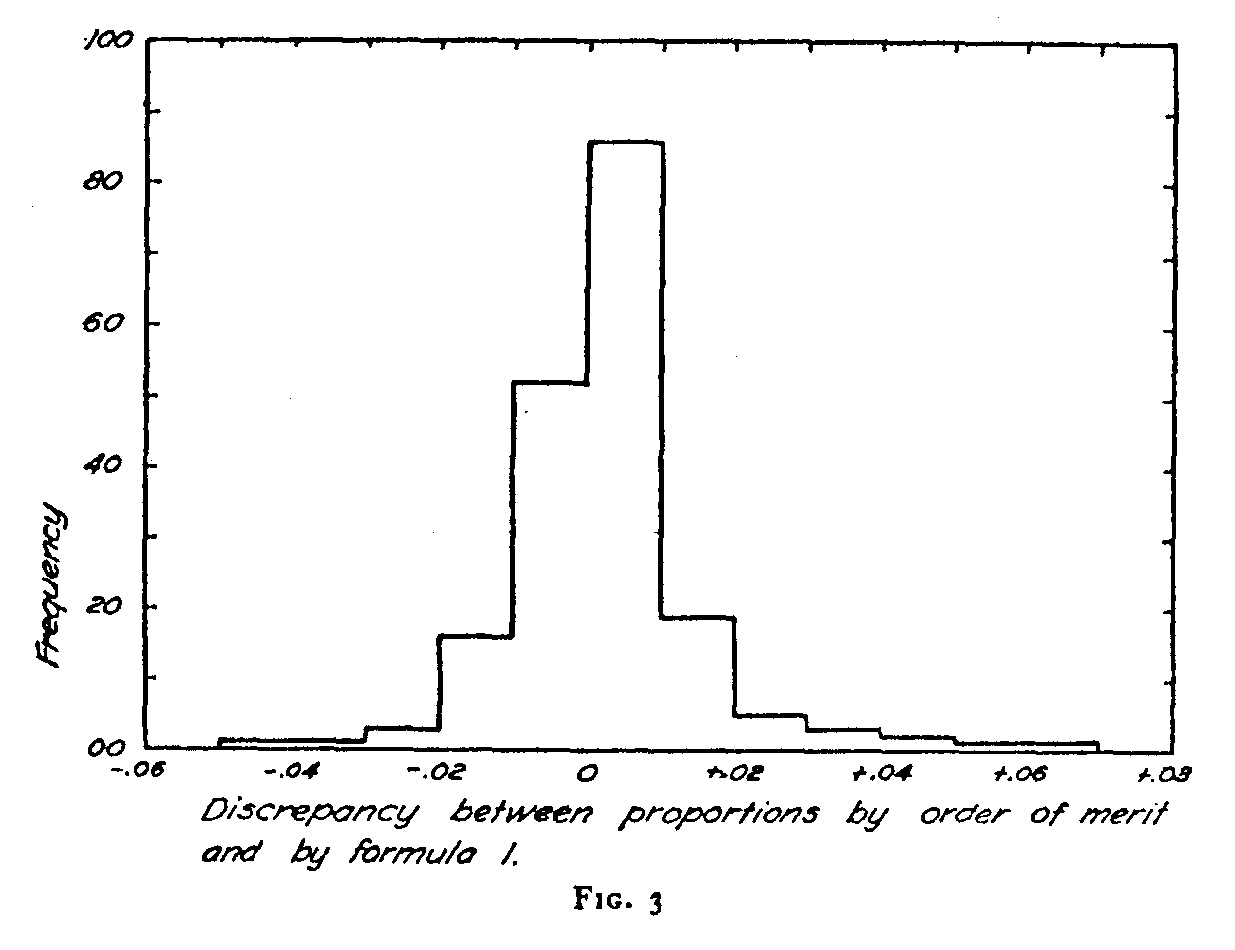sign, is .0078 which shows a very close agreement. The fact that the average discrepancy is less than one percent constitutes practical justification for equation (i) as a method of estimating the proportions of the constant method when the experimental procedure was that of simple rank order. The close similarity in results from the order of merit method and the constant method was demonstrated by Miss Hevner.
It is to be expected that the scale values for the twenty specimens determined by the two sets of proportions should be practically identical and this is shown in figure 3. We

( 200) have tabulated the scale values determined by Miss Hevner for the order of merit method and for the same raw data treated by the present formula. The agreement is practically perfect as shown in figure 4. Not only are the two scales comparable but the units are identical since the proportions themselves agree and consequently the slope of the plot in figure 4 is unity.An important psychophysical inference may be drawn from these experiments. In a previous paper I have assumed that the correlation between discriminal errors of two specimens that are being compared is zero. That is a fundamental assumption of the law of comparative judgment. At that time I did not see any clear method of testing that assumption separately from the other assumptions involved in the same

( 201) study. Since the equation of comparative judgment has been shown to fit experimental data for a wide variety of stimuli I have felt assured that the assumptions were justified. In the present data we find, however, a specific verification for the assumption that the correlational term of the equation of comparative judgment is zero. If it were not zero, then the probabilities involved in equation (1) would not be independent and consequently the product of the several probabilities would not tally with the experimentally observed proportion of judgments that constitute a compound event. Since the equation satisfies the experimentally observed frequencies with a degree of accuracy that is unusual in psychological work, we are justified in concluding that the several probabilities are truly independent and that therefore the correlational term in the law of comparative judgment is zero as has been previously assumed.

In Miss Hevner's study a comparison was made between the order of merit method and the constant method in its complete form, namely paired comparison. These two methods were shown to be identical when treated by the equation of comparative judgment. We have here shown that it is not necessary in the order of merit method to tabulate separately all of the n(n — 1) judgments for each subject that are implied in his arrangement of n stimuli in a single rank order. It is possible to estimate the proportions directly from a frequency table of rank orders for each specimen. This makes it possible to use simple rank order when that method is .experimentally the easiest and to extract from the rank order data the proportions that would be obtained by the laborious constant method or the even more laborious paired comparison method. Miss Hevner has previously shown that the order of merit method gives results practically identical with the constant method. We have also verified our previous assumption that the discriminal errors in a comparison of two stimuli are usually uncorrelated. This assumption enters the law of comparative judgment when the correlational term is assumed to be zero.